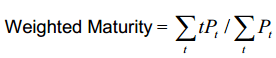# Effective Maturity in Basel II

Under the advanced IRB approach of the Basel II accord, the banks are allowed to develop their own internal models that they can use to measure the capital require for credit risk. Under this model, the banks are allowed to make their own estimation of various components including: the probability of default (PD), loss given default (LGD), exposure at default (EAD) and effective maturity (M).

The component Effective Maturity is referred to as M.

The effective maturity is the contractual maturity of the facility or the transaction which will be either.

1. M is calculated as the maximum remaining time (in years) that the obligor is permitted to take to fully discharge its contractual obligation, including principal, interest, and fees, under the terms of loan agreement.
2. For an instrument subject to a pre-determined, minimum amortisation schedule, the weighted maturity of the remaining minimum contractual principal payments, defined as:where Pt denotes the minimum amount of principal contractually payable t months in the future.

Effective maturity is measured as a Macaulay duration under the assumption that interest rates are zero.

A bank is required to calculate the effective maturity (M) for each wholesale exposure. The method of calculating effective maturity varies for different exposures.

Simple fixed exposures

For simple fixed wholesale exposures, M is the weighted-average remaining maturity (in years) of the expected contractual cash flows from the exposure, using the undiscounted amounts of the cash flows as weights.  The bank also has the choice of using the nominal remaining maturity of the exposure as effective maturity.

Exposures within a netting agreement

The transactions subject to a qualifying master netting agreement such as repo-style transactions, eligible margin loans, and OTC derivative contracts, the effective maturity is calculated as the weighted-average remaining maturity of the individual transactions subject to the qualifying master netting agreement, with the weight of each individual transaction equal to the notional amount of the transaction.

For most exposures, Basel II caps the effective maturity to five years and floors to one year.  If the original maturity of the exposure is less than 1 year and is not a part of a bank’s ongoing financing of the obligor, the effective maturity is subject to a floor equal to the largest minimum holding period of the transactions in the netting set. Examples of transactions include amounts due from other banks, bankers’ acceptances, and sovereign exposures.